Imagination is more important than knowledge... Albert Einstein

Guess is more important than calculation --- Knowhowacademy.com

Equation - System of Equations

For ACT
Number of Total Problems: 19.
FOR PRINT ::: (Book)

 Problem Num : 1 Type: None Section:Equation Theme:None Adjustment# : Difficulty: 1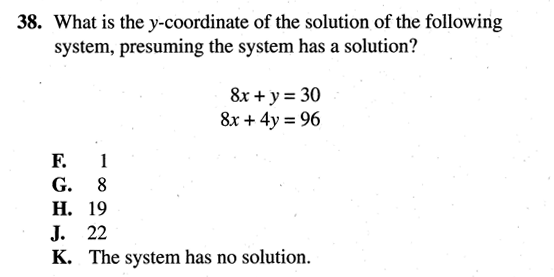Category System of Equations
Analysis

 Problem Num : 2 Type: None Section:Equation Theme:None Adjustment# : Difficulty: 1Category System of Equations
Analysis f+8r=200 f+10r=230

 Problem Num : 3 Type: None Section:Equation Theme:None Adjustment# : Difficulty: 1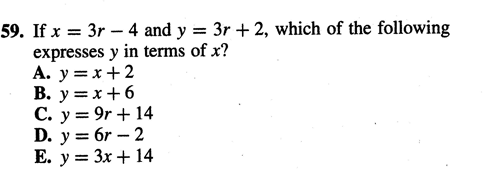Category System of Equations
Analysis

 Problem Num : 4 Type: None Section:Equation Theme:None Adjustment# : Difficulty: 1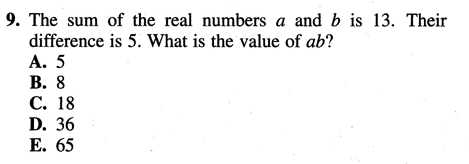Category System of Equations
Analysis

 Problem Num : 5 Type: None Section:Equation Theme:None Adjustment# : Difficulty: 1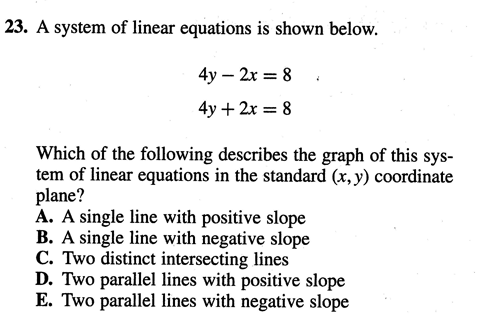Category System of Equations
Analysis

 Problem Num : 6 Type: None Section:Equation Theme:None Adjustment# : Difficulty: 1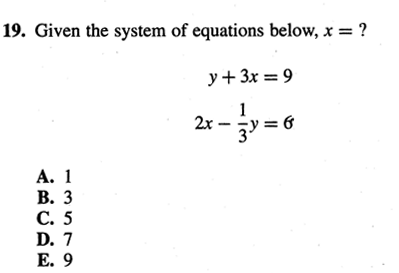Category System of Equations
Analysis

 Problem Num : 7 Type: None Section:Equation Theme:None Adjustment# : Difficulty: 1Category System of Equations
Analysis

 Problem Num : 8 Type: None Section:Equation Theme:None Adjustment# : Difficulty: 1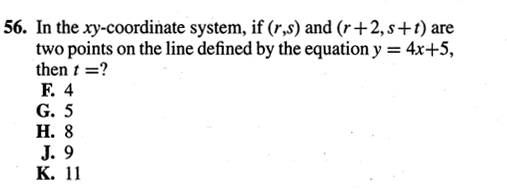Category System of Equations
Analysis

 Problem Num : 9 Type: None Section:Equation Theme:None Adjustment# : Difficulty: 1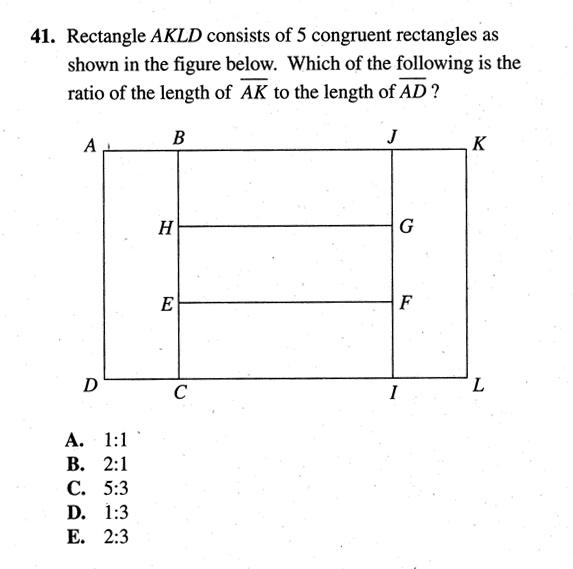Category System of Equations
Analysis AB=5ab AB=(a+2b)a a=3b

 Problem Num : 10 Type: None Section:Equation Theme:None Adjustment# : Difficulty: 1Category System of Equations
Analysis